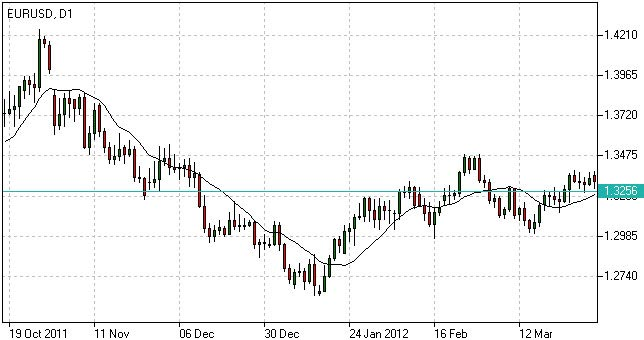# Moving Average Explained - What Is Moving Average

## Moving Average Definition

Moving Average is a technical analysis tool that shows average price over a given period of time, which is used to smoothen price fluctuations and therefore to determine trend direction and strength.

Test the Indicator in Action
Once opened Demo you will be supplied with educational materials and online support in your own language

Depending of the method of averaging, distinguish between simple moving average (SMA), smoothed moving average (SMMA) and exponential moving average (EMA).

## How to Use Moving Average

Generally moving average curves analysis includes the following principles:

• Direction of moving average curve reflects prevailing trend over a period;
• Low-period averaging may give more false signals, while large-period averaging tend to be lagging;
• To increase (decrease) sensitivity of the curve one should decrease (increase) the period of averaging;
• Average curves are more useful in trending environment.

Comparing moving average with price movements:

• A strong buy (sell) signal arise if price crosses from below (from above) its rising (falling) moving average curve;
• A weak buy (sell) signal arise if price crosses from below (from above) its falling (rising) moving average curve.

Comparing moving average curves of different periods:

• A rising (falling) lower-period curve crossing from below (above) another rising (falling) longer-period curve gives a strong buy (sell) signal;
• A rising (falling) lower-period curve crossing from below (above) another falling (rising) longer-period curve gives a weak buy (sell) signal.Moving Average (MA) Indicator

Moving average strategy is essentially a trend following means. Its objective is to signal the beginning of a new trend or a trend reversal. Herein, its main purpose is to track the progress of the trend and not to predict market action in the same sense that technical analysis attempts to do. By its nature, Moving Average is a follower; it follows the market telling that a new trend has begun or reversed only after the fact.

## Moving Average Formula (Calculation)

The calculation of the simple moving average is done through the method of calculating the average price over a given period of time.

```SMA = Sum (Close (i), N) / N,

where:
Close (i) – current close price;
N – period of averaging.
```

The calculation of the exponential moving average is done by taking into account the prices of the previous period:

```EMA(t) = EMA(t-1) + (K x [Close(t) – EMA(t-1)]),

where:
t – current period;K = 2 / (N + 1), N – period of averaging.
```